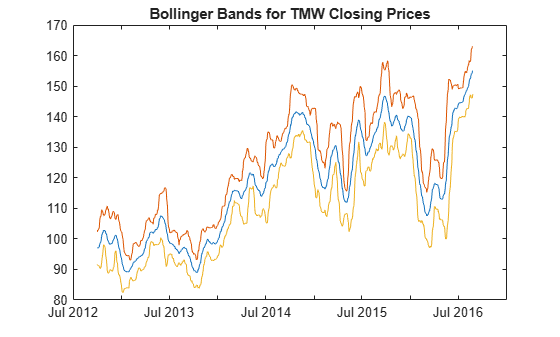Documentation

# bollinger

Time series Bollinger band

`bollinger` has been partially removed and will no longer accept a `fints` object (`tsobj`) argument. Use a matrix, `timetable`, or `table` instead for financial time series.

Use `fts2timetable` to convert a `fints` object to a `timetable` object.

## Syntax

``[middle,upper,lower] = bollinger(Data)``
``[middle,upper,lower] = bollinger(___,Name,Value)``

## Description

example

````[middle,upper,lower] = bollinger(Data)` calculates the middle, upper, and lower bands that make up the Bollinger bands from a series of data. A Bollinger band chart plots actual asset data along with three other bands of data: the upper band that is two standard deviations above a user-specified moving average; the lower band that is two standard deviations below that moving average; and the middle band that is the moving average itself.```

example

````[middle,upper,lower] = bollinger(___,Name,Value)` adds optional name-value pair arguments. ```

## Examples

collapse all

Load the file `SimulatedStock.mat`, which provides a timetable (`TMW`) for financial data for TMW stock.

```load SimulatedStock.mat [middle,upper,lower]= bollinger(TMW); CloseBolling = [middle.Close, upper.Close,... lower.Close]; plot(middle.Time,CloseBolling) title('Bollinger Bands for TMW Closing Prices')```## Input Arguments

collapse all

Data for market prices, specified as a matrix, table, or timetable. For matrix input, `Data` must be column-oriented.

Data Types: `double` | `table` | `timetable`

### Name-Value Pair Arguments

Specify optional comma-separated pairs of `Name,Value` arguments. `Name` is the argument name and `Value` is the corresponding value. `Name` must appear inside quotes. You can specify several name and value pair arguments in any order as `Name1,Value1,...,NameN,ValueN`.

Example: ```[middle,upper,lower] = bollinger(TMW_CLOSE,'WindowSize',10,'Type',1)```

Number of observations of the input series to include in the moving average in periods, specified as the comma-separated pair consisting of `'WindowSize'` and a scalar positive integer.

Data Types: `double`

Type of moving average to compute, specified as the comma-separated pair consisting of `'Type'` and a scalar integer with a value of `0` (simple) or `1` (linear).

Data Types: `double`

Number of standard deviations for the upper and lower bounds, specified as the comma-separated pair consisting of `'NumStd'` and a scalar positive integer.

Data Types: `double`

## Output Arguments

collapse all

Moving average series representing the middle band, returned with the same number of rows (`M`) and the same type (matrix, table, or timetable) as the input `Data`.

Moving average series representing the upper band, returned with the same number of rows (`M`) and the same type (matrix, table, or timetable) as the input `Data`.

Moving average series representing the lower band, returned with the same number of rows (`M`) and the same type (matrix, table, or timetable) as the input `Data`.

 Achelis, S. B. Technical Analysis from A to Z. Second Edition. McGraw-Hill, 1995, pp. 72–74.